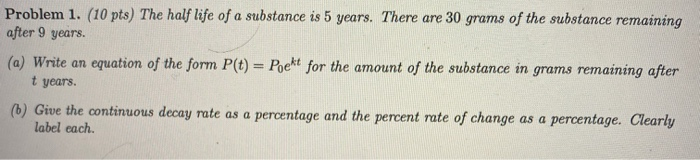# Problem 1. (10 pts) The half life of a substance is 5 years. There are 30...

###### Question:Problem 1. (10 pts) The half life of a substance is 5 years. There are 30 grams of the substance remaining after 9 years. (a) Write an equation of the form P(t) = Poekt for the amount of the substance in grams remaining after t years. (6) Give the continuous decay rate as a percentage and the percent rate of change as a percentage. Clearly label each.